This post is meant to show you how to apply Robust Tensor PCA in practice using TensorLy. I'll provide a little bit of mathematical background on Robust PCA, feel free to skip that parts if Math is not your cup of tea. Otherwise, grab some biscuits. Then I'll demonstrate how to easily apply Robust Tensor PCA on images using TensorLy.

# A short introduction to robust PCA¶

This section is here to give a little bit of background on Robust PCA: you do not need this for the rest of the article, only read if you are interested!

In short, Robust PCA is analogous to traditional PCA but instead of recovering a low rank approximation of the matrix under some Gaussian noise assumption, it decomposes it as the sum of of a low rank matrix and a sparse one. Tensor Robust PCA further generalises this notion to tensors.

Let's start with traditional Principal Component Analysis. There are several ways to formulate the PCA problem, here we show the minimum reconstruction error formulation. Formally, we want to minimise the Frobenius norm of the reconstruction error under orthogonality constraint over the projection weights. Let $X \in \mathbb{R}^{M, N}$ be an $N$ by $M$ matrix. We want to find the orthonormal projection matrix $W \in \mathbb{R}^{M, K}, K \leq M$ that solves:

\begin{equation*} \begin{aligned} & \min_{W} & & \|X - XWW^T \|_F^2\\ & \text{subject to} & & W^TW = Id\\ \end{aligned} \end{equation*}

If we write $D$ the reconstructed matrix $D = XWW^T$, we can equivalently write the problem as:

\begin{equation*} \begin{aligned} & \min_{W} & & \|X - D\|_F^2\\ & \text{subject to} & & rank(D) \leq K\\ \end{aligned} \end{equation*}

Robust PCA still wants to minimise the error but this time under the asumption that the noise is gross but sparse: instead of minimising an $\ell_2$ norm, we minimise an $\ell_0$ norm of the reconstruction error. In addition, instead of setting an upper bound on the rank of the reconstruction, we directly seek to minimze it:

\begin{equation*} \begin{aligned} & \min_{D} & & rank(D) + \|X - D\|_0\\ \end{aligned} \end{equation*}

However, this problem is NP-hard, which means it cannot be solved in Polynomial time (i.e. as the size of your data increases, the time it takes to solve it increases exponentially).

A nice way around this is to relax the problem using the convex surrogate of the norm $\ell_0$ and of the rank operator as proposed by Cantes in his paper "Robust Principal Component Analysis" in ACM Journal, 2011.

This turns the problem into a tractable one:

\begin{equation*} \begin{aligned} & \min_{\tilde D} & & \|D\|_* + \lambda \|X - D\|_1 \\ \end{aligned} \end{equation*}

Note that I have cheekily added a regularisation parameter on the norm $\ell_1$ of the error while you weren't looking: in practice, this parameter controls how sparse the error matrix will be and needs to be tuned to each particular data.

Robust Tensor PCA generalises this problem to tensors. Instead of minimizing the nuclear norm of the data tensor $\tilde{X}$, we minimise the nuclear norm of its unfoldings, $\tilde{X_{[i]}}$ along each mode $i, i \in \left[1, \cdots, N\right].$

In other words, the problem robust tensor PCA solves is:

\begin{equation*} \begin{aligned} & \min_{\tilde D} & & \sum_{i=1}^N \|\tilde D_{[i]}\|_* + \lambda \|\tilde X - \tilde D\|_1 \\ \end{aligned} \end{equation*}

This is implemented in TensorLy using the Alternating Direction Method of Multipliers (ADMM) and wraped in a nice API.

# Example on the YaleB dataset¶

Here we will apply Robust Tensor PCA on the YaleB dataset. It consists images of the faces of several people taken from the same angle but with different illumniations. We will use the cropped version where the images have been cropped to contain only the faces.

## Getting the data¶

I wrote a convenience function to download the data if it hasn't been done already and load it into a dictionary you can easily use:

In :
from pathlib import Path
from urllib.request import urlretrieve
import zipfile
from scipy.ndimage.interpolation import zoom#
import numpy as np

def fetch_cropped_yaleb(data_folder, zooming=0.5, max_n_subjects=None):
"""Returns a dictionary of paths

Parameters
----------
data_folder: string
zooming: float, optional, default is 0.5
factor by which to resize the images
max_n_subjects: {None, int}, optional, default is None
if not None, only the first max_n_subjects are returned

Returns
-------
dict: {
subjects_1: {'images': [image_1, ... image_N],
'ambient': image_ambient,
}
}

images are stored as numpy arrays
"""
url = 'http://vision.ucsd.edu/extyaleb/CroppedYaleBZip/CroppedYale.zip'
yaleb_path = Path(data_folder).joinpath('cropped_yaleb')

if not yaleb_path.joinpath('CroppedYale').exists():
yaleb_path.mkdir(parents=True)

# If not already unzip, do it
if not list(yaleb_path.iterdir()):
zip_path = yaleb_path.joinpath('yaleb.zip')

if not zip_path.exists():
urlretrieve(url, zip_path.as_posix())

zfile = zipfile.ZipFile(zip_path.as_posix())
zfile.extractall(path=yaleb_path.as_posix())

yaleb = {}
for folder_path in yaleb_path.joinpath('CroppedYale').iterdir():
if max_n_subjects is not None and len(yaleb) > max_n_subjects:
return yaleb

if not folder_path.is_dir():
continue

video_name = folder_path.name
paths = sorted(list(folder_path.glob('*.pgm')))
images = []
for path in paths:
if 'Ambient' in path.name:
else:

data = {'images':np.concatenate(images),
'ambient':ambient}
yaleb[video_name] = data

return yaleb


All you need is define where you want to save the data on your disk and the function will download and unzip it for you:

In [ ]:
dataset_path = '/data/tensorly_data/'

data = fetch_cropped_yaleb(dataset_path, zooming=0.3, max_n_subjects=5)


## Create the data tensor¶

First we accumulate all the data into a tensor: each image is in grayscale and represented as a 2-dimensional numpy array of shape (height, width). We will stack them in a tensor of size (n_images, height, width):

In :
X = np.concatenate([data[key]['images'] for key in data], axis=0)

print(X.shape)

(384, 58, 50)


Optionally, one might want to normalise the values of the images (they are currently uint8 ranging from 0 to 255) so let's convert them into float64:

In :
X = X.astype(np.float64)
X -= X.mean()


## Visualise the data¶

Let's define a simple helper function to visualise the images:

In :
import matplotlib.pyplot as plt
%matplotlib inline

def visualise_images(X, n_images, n_columns, randomise=True):
indices = np.arange(X.shape)
np.random.shuffle(indices)
indices = indices[:n_images]
cmap = plt.cm.Greys_r
n_rows = np.ceil(n_images / n_columns)
fig = plt.figure(figsize=(2*n_columns, 2*n_rows))
fig.subplots_adjust(left=0, right=1, bottom=0, top=1, hspace=0.05, wspace=0.05)

# plot the digits: each image is 8x8 pixels
for i, e in enumerate(indices):
ax = fig.add_subplot(n_rows, n_columns, i + 1, xticks=[], yticks=[])
ax.imshow(X[e], cmap=cmap, interpolation='nearest')

In :
visualise_images(X, 12, 4)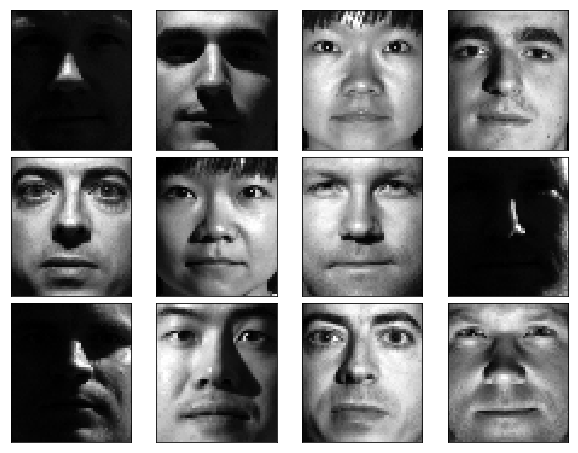## Add salt and pepper noise¶

To test the Robust PCA and its ability to recover the low rank structure under gross, sparse error, we will add some noise to the images using TensorLy's function add_noise:

In :
from tensorly.random.noise import add_noise
from tensorly.random import check_random_state

random_state = 1234


Note that we define a random_state to fix the seed of the random generator and allow for reproducible results. Feel free to change the state or remove this altogether.

In :
X = add_noise(X, noise='salt_pepper', percent=0.15, inplace=True, random_state=random_state)

In :
visualise_images(X, 12, 4)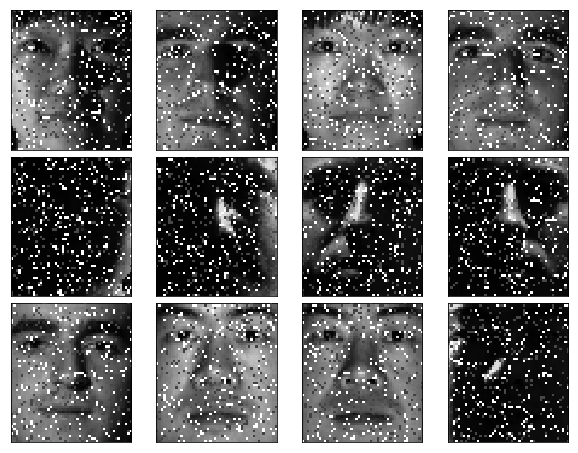## Apply Robust PCA¶

Robust PCA decomposes the data tensor (X) into the sum of a low rank tensor (in this case, a denoised version) and a sparse tensor (the noise). In TensorLy, you can directly use the :function:tensorly.decomposition.robust_pca fuction.

In :
from tensorly.decomposition import robust_pca


The robust_pca functions returns two tensors with identical dimensions to the tensor decomposed: the low rank part and the sparse part:

In :
low_rank_part, sparse_part = robust_pca(X, reg_E=0.04, learning_rate=1.2, n_iter_max=20)


You can check that both term are tensor with the same shape as X:

In :
print('X.shape={} == low_rank_part.shape={} == sparse_part.shape={}.'.format(X.shape, low_rank_part.shape, sparse_part.shape))

X.shape=(384, 58, 50) == low_rank_part.shape=(384, 58, 50) == sparse_part.shape=(384, 58, 50).


## Visualise the result¶

Again let's write a simple helper function to visualise the results of the Robust Tensor PCA:

In :
def visualise_rpca(X, low_rank_part, sparse_part, n_images=10):
"""A little helper function to visualise the result of tensor RPCA
"""
indices = np.arange(X.shape)
np.random.shuffle(indices)
indices = indices[:n_images]

fig = plt.figure(figsize=(10, 2*n_images))
fig.subplots_adjust(left=0, right=1, bottom=0, top=1, hspace=0.05, wspace=0.05)

# plot the digits: each image is 8x8 pixels
for i, e in enumerate(indices):
cmap = plt.cm.Greys_r

ax = fig.add_subplot(n_images, 4, 4*i + 1, xticks=[], yticks=[])
ax.imshow(X[e], cmap=cmap, interpolation='nearest')
if not i:
ax.set_title('Original')

ax = fig.add_subplot(n_images, 4, 4*i + 2, xticks=[], yticks=[])
ax.imshow(low_rank_part[e], cmap=cmap, interpolation='nearest')
if not i:
ax.set_title('Low-rank')

ax = fig.add_subplot(n_images, 4, 4*i + 3, xticks=[], yticks=[])
ax.imshow(sparse_part[e], cmap=cmap, interpolation='nearest')
if not i:
ax.set_title('Sparse')

ax = fig.add_subplot(n_images, 4, 4*i + 4, xticks=[], yticks=[])
ax.imshow(low_rank_part[e]+sparse_part[e], cmap=cmap, interpolation='nearest')
if not i:
ax.set_title('Reconstruction')

In :
visualise_rpca(X, low_rank_part, sparse_part, n_images=6)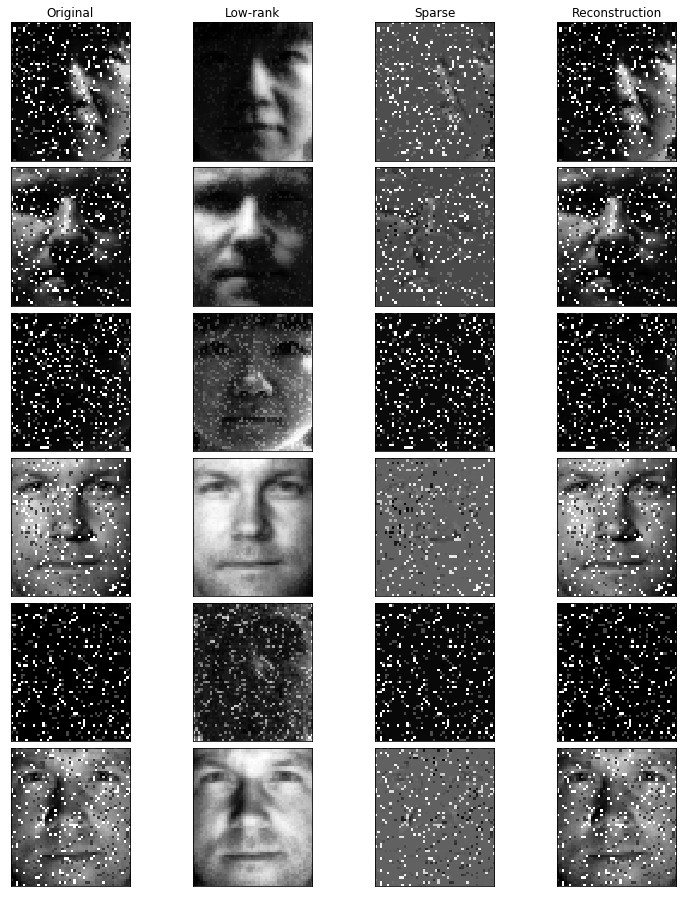As you can see, the Robust PCA was able to isolate the clean tensor from the noise.

Let's now add some missing values. We will replace the pixels in random patches of size 20 by 20:

In :
from tensorly.random.noise import patch_noise


We will create a mask with the same shape as our data tensor which will have value one for pathces of size (20, 20) position randomly in each sample:

In :
noise_mask = patch_noise(np.zeros(X.shape), (20, 20), noise=1, inplace=True, random_state=random_state).astype(bool)


Set the pixels inside the patches to zero:

In :
X[noise_mask] = 0


Now, let's check the result:

In :
visualise_images(X, 12, 4)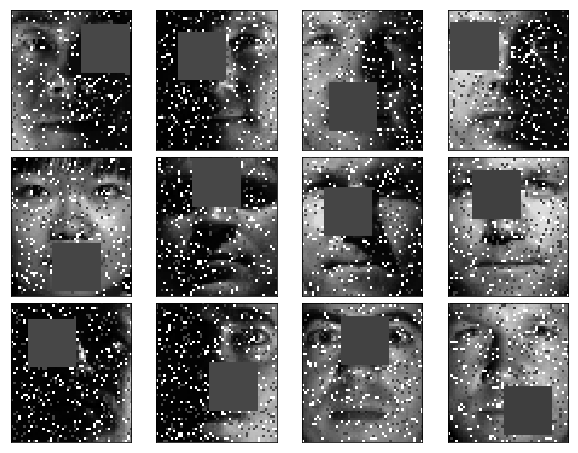The robust_pca function can take a mask as a parameter that is 0/False wherever the values are missing and 1/True everywhere else.

In :
low_rank_part, sparse_part = robust_pca(X, mask=~noise_mask, reg_E=0.04, learning_rate=1.2, n_iter_max=20)


Let's visualise the results:

In :
visualise_rpca(X, low_rank_part, sparse_part, n_images=6)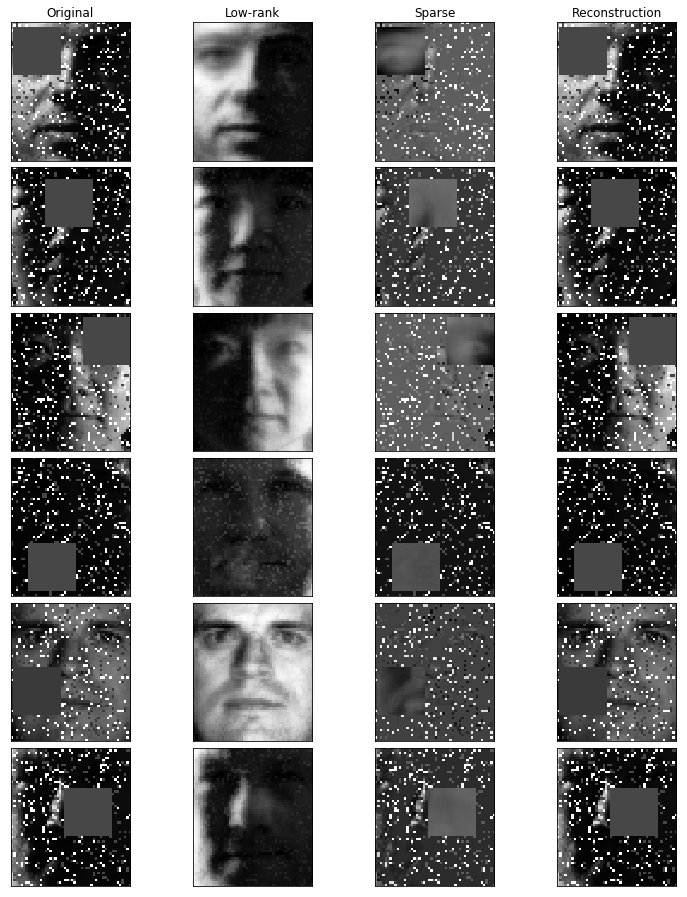Once again the Robust Tensor PCA was able to isolate the gross error from the rest.

# Example on the Olivetti dataset¶

It seems that the website I had used to get the YaleB data is down, so as a backup data, we are going to use the Olivetti dataset. The excellent scikit-learn has utility function to download various datasets, including the one we are interested in:

In :
from sklearn.datasets import fetch_olivetti_faces


We are going to use directly the images instead of the vectorized version:

In :
data = fetch_olivetti_faces()
# We directly use the images as our data tensor
X = data.images

X = X.astype(np.float64)
X -= X.mean()

In :
visualise_images(X, 12, 4)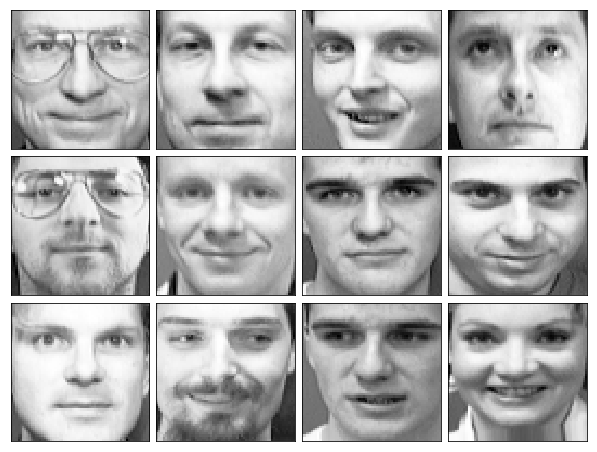As previously, we add some noise and missing values:

In :
X = add_noise(X, noise='salt_pepper', percent=0.15, inplace=False, salt_value=1, pepper_value=1, random_state=random_state)
noise_mask = patch_noise(np.zeros(X.shape), (20, 20), noise=1, inplace=True, random_state=random_state).astype(bool)

In :
visualise_images(X, 12, 4)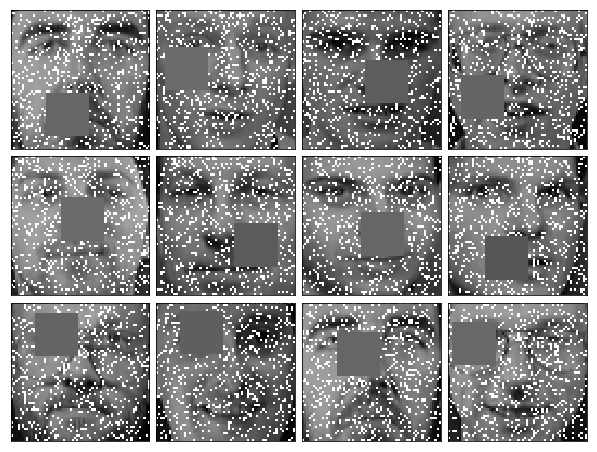Let's apply Robust PCA on this mess:

In :
low_rank_part, sparse_part = robust_pca(X, mask=~noise_mask, reg_E=0.04, learning_rate=1.2, n_iter_max=50)

In :
visualise_rpca(X, low_rank_part, sparse_part, n_images=6)